# IB DP Physics: HL复习笔记9.2.2 Intensity of Interference Maxima & Minima

### Intensity of Interference Maxima & Minima

• Using different sources of monochromatic light demonstrate that:
• Increasing the wavelength increases the width of the fringes
• The angle of diffraction of the first minima can be found using the equation:
•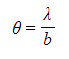•  Where:
• θ = the angle of diffraction (radians)
• λ = wavelength (m)
• b = slit width (m)
• This equation explains why red light produces wider maxima
• It is because the longer the wavelength, λ, the larger the angle of diffraction, θ
• It also explains the coloured fringes seen when white light is diffracted
• It is because red light (longer λ) will diffract more than blue light (shorter λ)
• This creates fringes which are blue nearer the centre and red further out
• It also explains why wider slits cause the maxima to be narrower
• It is because the wider the slit, b, the smaller the angle of diffraction, θ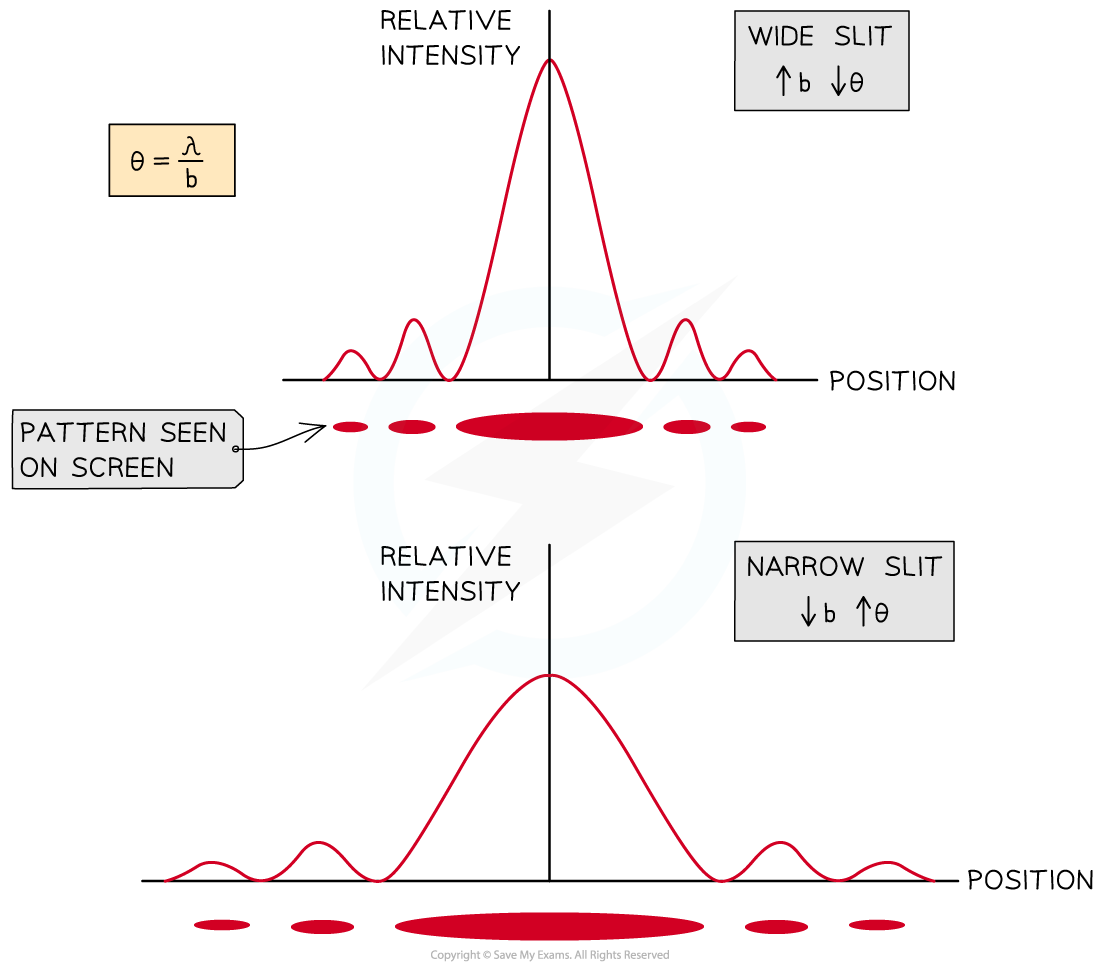Slit width and angle of diffraction are inversely proportional. Increasing the slit width leads to a decrease in angle of diffraction, hence the maxima appear narrower

#### Single Slit Geometry

• The diffraction pattern made by waves passing through a slit of width b can be observed on a screen placed a large distance away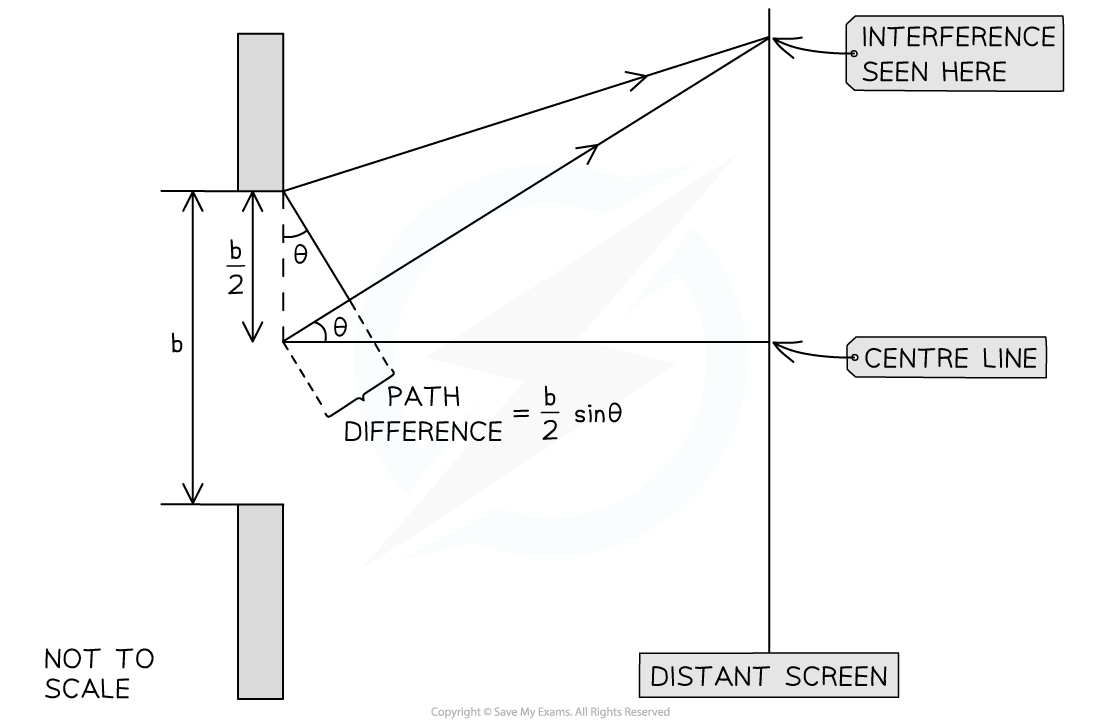The geometry of single-slit diffraction

####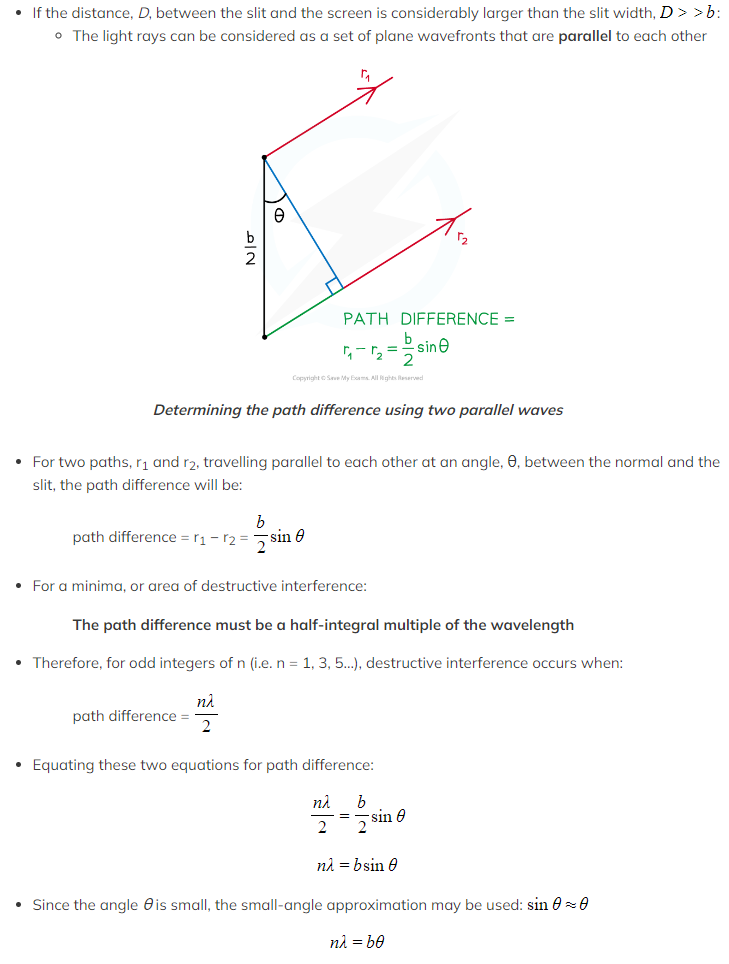Worked Example

A group of students are performing a diffraction investigation where a beam of coherent light is incident on a single slit with width, b.

The light is then incident on a screen which has been set up a distance, D, away.A pattern of light and dark fringes is seen.

The teacher asks the students to change their set-up so that the width of the first bright maximum increases.

Suggest three changes the students could make to the set-up of their investigation which would achieve this.

Step 1: Write down the equation for the angle of diffraction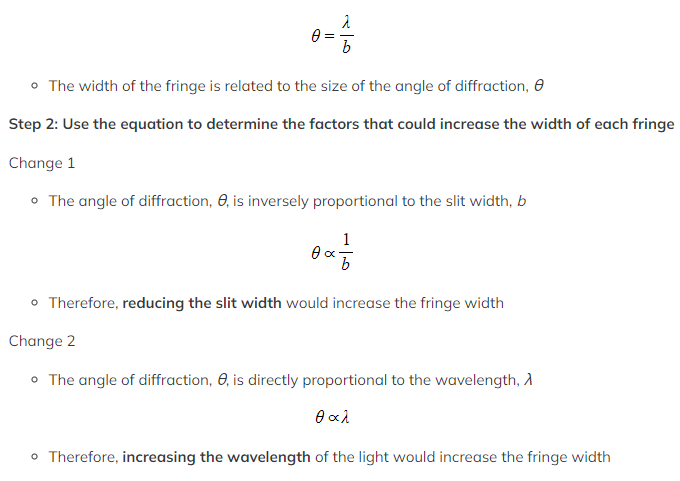Change 3

• The distance between the slit and the screen will also affect the width of the central fringe
• A larger distance means the waves must travel further hence, will spread out more
• Therefore, moving the screen further away would increase the fringe width